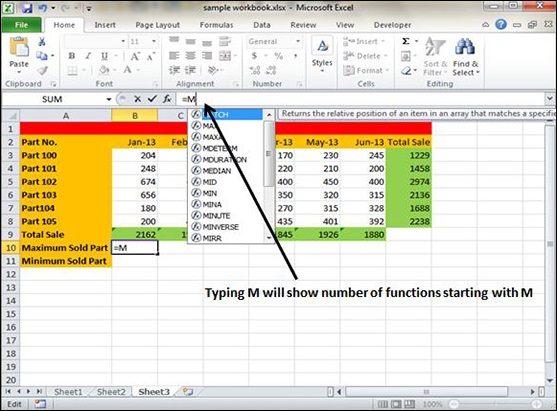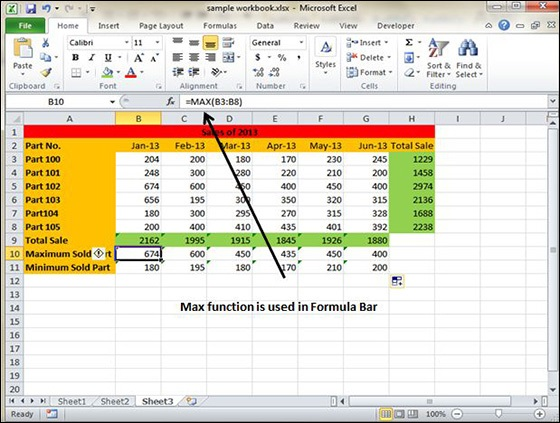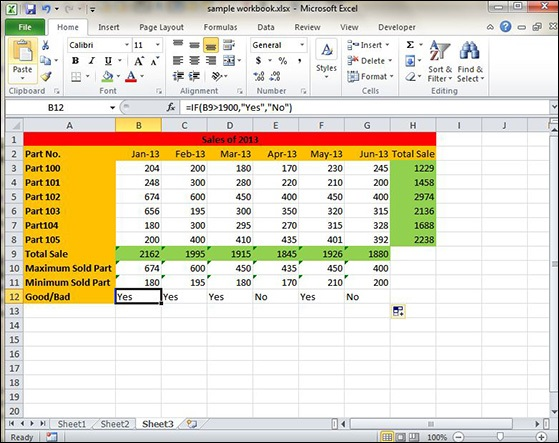# Using Functions in Excel 2010

## Functions in Formula

Many formulas you create use available worksheet functions. These functions enable you to greatly enhance the power of your formulas and perform calculations that are difficult if you use only the operators. For example, you can use the LOG or SIN function to calculate the Logarithm or Sin ratio. You can’t do this complicated calculation by using the mathematical operators alone.

## Using Functions

When you type = sign and then type any alphabet you will see the searched functions as below.Suppose you need to determine the largest value in a range. A formula can’t tell you the answer without using a function. We will use formula that uses the MAX function to return the largest value in the range B3:B8 as =MAX(A1:D100).Another example of functions. Suppose you want to find if the cell of month is greater than 1900 then we can give Bonus to Sales representative. The we can achieve it with writing formula with IF functions as =IF(B9>1900,"Yes","No")## Function Arguments

In the above examples, you may have noticed that all the functions used parentheses. The information inside the parentheses is the list of arguments.

Functions vary in how they use arguments. Depending on what it has to do, a function may use.

• No arguments − Examples − Now(), Date(), etc.

• One argument − UPPER(), LOWER(), etc.

• A fixed number of arguments − IF(), MAX(), MIN(), AVERGAGE(), etc.

• Infinite number of arguments

• Optional arguments